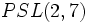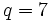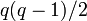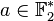# Element structure of projective special linear group:PSL(3,2)

This article gives specific information, namely, element structure, about a particular group, namely: projective special linear group:PSL(3,2).
View element structure of particular groups | View other specific information about projective special linear group:PSL(3,2)

This article describes the element structure of projective special linear group:PSL(3,2), which is the same as$SL(3,2)$,$GL(3,2)$. It is also isomorphic to the projective special linear group of degree two over field:F7, i.e., the group$PSL(2,7)$.

## Summary

Item Value
order of the whole group (total number of elements) 168
conjugacy class sizes 1,21,24,24,42,56
in grouped form: 1 (1 time), 21 (1 time), 24 (2 times), 42 (1 time), 56 (1 time)
maximum: 56, number of conjugacy classes: 6, lcm: 168
order statistics 1 of order 1, 21 of order 2, 56 of order 3, 42 of order 4, 48 of order 7
maximum: 7, lcm (exponent of the whole group): 84

## Conjugacy class structure

### Interpretation as projective special linear group of degree two

Compare with element structure of projective special linear group of degree two over a finite field#Conjugacy class structure

We consider the group as$PSL(2,q)$,$q = 7$. We use the letter$q$ to denote the generic case of$q \equiv 3 \pmod 4$.

Nature of conjugacy class upstairs in$SL_2$ Eigenvalues Characteristic polynomial Minimal polynomial Size of conjugacy class (generic$q$ that is 3 mod 4) Size of conjugacy class ($q = 7$) Number of such conjugacy classes (generic$q$ that is 3 mod 4) Number of such conjugacy classes ($q = 7$) Total number of elements (generic$q$ that is 3 mod 4) Total number of elements ($q = 7$)
Diagonalizable over$\mathbb{F}_q$ with equal diagonal entries, hence a scalar$\{ 1,1 \}$ or$\{ -1,-1\}$, both correspond to the same element$(x - a)^2$ where$a \in \{ -1,1 \}$$x - a$ where$a \in \{ -1,1\}$ 1 1 1 1 1 1
Diagonalizable over$\mathbb{F}_{q^2}$, not over$\mathbb{F}_q$, eigenvalues square roots of$-1$ Square roots of$-1$$x^2 + 1$$x^2 + 1$$q(q - 1)/2$ 21 1 1$q(q - 1)/2$ 21
Not diagonal, has Jordan block of size two$1$ (multiplicity 2) or$-1$ (multiplicity 2). Each conjugacy class has one representative of each type.$(x - a)^2$ where$a \in \{ -1,1 \}$$x - a$ where$a \in \{ -1,1\}$$(q^2 - 1)/2$ 24 2 2$q^2 - 1$ 48
Diagonalizable over$\mathbb{F}_{q^2}$, not over$\mathbb{F}_q$. Must necessarily have no repeated eigenvalues. Eigenvalues not square roots of$-1$. Pair of conjugate elements of$\mathbb{F}_{q^2}$ of norm 1, not square roots of -1. Each pair identified with its negative pair.$x^2 - ax + 1$,$a \ne 0$ irreducible; note that$x^2 - ax + 1$'s pair and$x^2 + ax +1$'s pair get identified. Same as characteristic polynomial$q(q - 1)$ 42$(q - 3)/4$ 1$q(q - 1)(q - 3)/4$ 42
Diagonalizable over$\mathbb{F}_q$ with distinct (and hence mutually inverse) diagonal entries$\lambda, 1/\lambda$ where$\lambda \in \mathbb{F}_q \setminus \{ 0,1,-1,i,-i \}$ where$i,-i$ are square roots of$-1$. Note that the representative pairs$\{ \lambda, 1/\lambda \}$ and$\{ -\lambda,-1/\lambda \}$ get identified.$x^2 - (\lambda + 1/\lambda)x + 1$, again with identification.$x^2 - (\lambda + 1/\lambda)x + 1$, again with identification.$q(q + 1)$ 56$(q - 3)/4$ 1$q(q + 1)(q - 3)/4$ 56
Total NA NA NA NA NA$(q + 5)/2$ 6$(q^3 - q)/2$ 168

### Interpretation as general linear group of degree three over field:F2

Compare with element structure of general linear group of degree three#Conjugacy class structure. Since many cases (namely, those that rely on distinct eigenvalues) do not arise over field:F2, the cases have been omitted in the table below.

Nature of conjugacy class Eigenvalues Characteristic polynomial Minimal polynomial Size of conjugacy class Number of such conjugacy classes Total number of elements Semisimple? Diagonalizable over$\mathbb{F}_q$?
Diagonalizable over$\mathbb{F}_2$ with equal diagonal entries, hence a scalar$\{1,1,1 \}$$(x - 1)^3$$x - 1$ 1 1 1 Yes Yes
Diagonalizable over$\mathbb{F}_8$, not over$\mathbb{F}_2$ Distinct Galois conjugate triple of elements in$\mathbb{F}_{8}^\ast$. If one of the elements is$a$, the other two are$a^2$ and$a^2$. irreducible degree three polynomial over$\mathbb{F}_q$ same as characteristic polynomial 24 2 48 Yes No
One eigenvalue is in$\mathbb{F}_q^\ast$, the other two are in$\mathbb{F}_4 \setminus \mathbb{F}_q$ one element of$\mathbb{F}_2^\ast$, pair of Galois conjugates over$\mathbb{F}_2$ in$\mathbb{F}_4$. product of linear polynomial and irreducible degree two polynomial over$\mathbb{F}_q$ same as characteristic polynomial 56 1 56 Yes No
Has Jordan blocks of sizes 2 and 1 with equal eigenvalues over$\mathbb{F}_2$$\{ 1,1,1 \}$ with$a \in \mathbb{F}_2^\ast$$(x - 1)^3$$(x - 1)^2$ 42 1 42 No No
Has Jordan block of size 3$\{ a,a,a \}$ with$a \in \mathbb{F}_2^\ast$$(x - 1)^3$ same as characteristic polynomial 21 1 21 No No
Total NA NA NA NA 6 168 NA NA